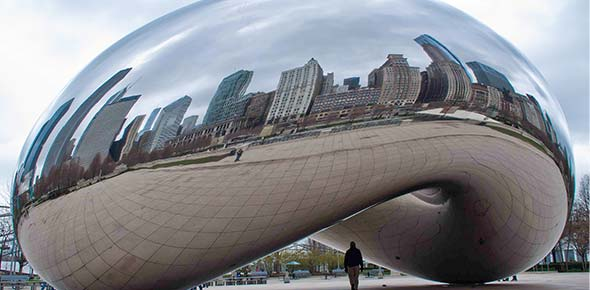# Chicago Electrical Exam

123 Questions | Total Attempts: 1586SettingsChicago Electrical Contractor Prep-Exam

• 1.
A motor draws 165 amps at a voltage of 460 with a feeder ft. length of 190. The copper wire (75 degree C) size desired is #2/0, what would the voltage drop be?
• A.

4.4

• B.

5.7

• C.

6.5

• 2.
A motor draws 83 amps at a voltage of 460 with a feeder ft. length of 190. The copper wire (75 degree C) size desired is #3, what would the voltage drop be?"
• A.

5.9

• B.

7.2

• C.

8.0

• 3.
A motor draws 21amps at a voltage of 230 with a feeder ft. length of 160. The copper wire (75 degree C) size desired is #10, Which minimum size copper wire is permissible by code to use?"
• A.

#10

• B.

#8

• C.

#6

• 4.
A motor draws 32 amps at a voltage of 230 with a feeder ft. length of 150. The copper wire (75 degree C) size desired is #8, Which minimum size copper wire is permissible by code to use?"
• A.

#8

• B.

#6

• C.

#4

• 5.
A motor draws 85 amps at a voltage of 230 with a feeder ft. length of 160. The copper wire (75 degree C) size desired is #3, Which minimum size copper wire is permissible by code to use?
• A.

3

• B.

2

• C.

1

• 6.
A motor draws 21 amps at a voltage of 460 with a feeder ft. length of 190. The copper wire (75 degree C) size desired is #10, Which minimum size copper wire is permissible by code to use?
• A.

#10

• B.

#8

• C.

#6

• 7.
A motor draws 47 amps at a voltage of 460 with a feeder ft. length of 190. The copper wire (75 degree C) size desired is #8, Which minimum size copper wire is permissible by code to use?
• A.

#8

• B.

#6

• C.

#4

• 8.
A motor draws 165 amps at a voltage of 460 with a feeder ft. length of 190. The copper wire (75 degree C) size desired is #2/0, Which minimum size copper wire is permissible by code to use?
• A.

1/0

• B.

2/0

• C.

3/0

• 9.
A motor draws 83 amps at a voltage of 460 with a feeder ft. length of 190. The copper wire (75 degree C) size desired is #3, Which minimum size copper wire is permissible by code to use?
• A.

3

• B.

2

• C.

1

• 10.
For purpose of computing service loads, the voltages not to be used is, 120/220V
• A.

True

• B.

False

• 11.
The unit lighting load that is specified for, occupancy use in the code book constitutes the required lighting load for each square foot (0.093 mz) of floor area.
• A.

True

• B.

False

• 12.
Each floor area of a building, dwelling unit, or other area involved shall be, computed from the interior wall dimensions to determine the total square footage for, service load calculations.
• A.

True

• B.

False

• 13.
The computed floor area of dwelling units, shall include open or enclosed porches.
• A.

True

• B.

False

• 14.
In all occupancies, what is the minimum design load to include for, each general purpose outlets.
• A.

120 volt-amperes

• B.

180 volt-amperes

• C.

1500 volt-amperes

• 15.
Outlets serving switchboards, and switchgear frames in telephone exchanges need not be included, in minimum design load computations.
• A.

True

• B.

False

• 16.
An outlet for a specific appliance not covered in Article 220 shall have the computed ampere rating based on what?
• A.

Appliance rated amperes

• B.

180 volt-amperes for outlet

• C.

2000 watts per appliance

• 17.
What is the minimum Volt-Amperes per Square Foot of, General Lighting Load for a Barber shop that, you can use?
• A.

2

• B.

3

• C.

3 1/2

• 18.
What is the minimum Volt-Amperes per Square Foot of, General Lighting Load for, a Hospital that you can use?
• A.

2

• B.

3

• C.

3 1/2

• 19.
What is the minimum Volt-Amperes per Square Foot of, General Lighting Load for a School that, you can use?
• A.

2

• B.

3

• C.

3 1/2

• 20.
What is the minimum Volt-Amperes per Square Foot of, General Lighting Load for an auditorium that, you can use?
• A.

1

• B.

2

• C.

3

• 21.
What is the minimum Volt-Amperes per Square Foot of, General Lighting Load for a Court room that, you can use?
• A.

2

• B.

3

• C.

3 1/2

• 22.
The volt-amperes of outlets for heavy duty lamp holders shall be computed at a minimum of,
• A.

180

• B.

120

• C.

600

• 23.
When a service voltage exceeds 15,000 volts between conductors enter a building, they shall terminate in a?
• A.

Metal enclosed switchgear compartment

• B.

A transformer vault

• C.

A metal enclosed switchgear compartment or a transformer vault

• 24.
Additional building services, with a supply voltage of 600 volts or less, are permitted when the capacity requirements are in excess of how many amperes?
• A.

2000

• B.

3000

• C.

4000

• 25.
A service supplying a building or other structure can not pass through the interior of another building or other structure.
• A.

True

• B.

False

Related TopicsBack to top